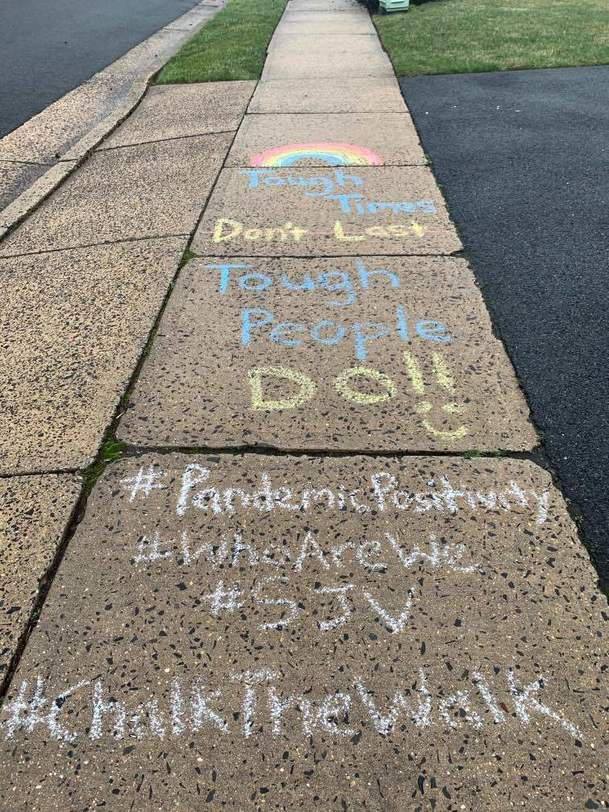# Mrs. Perona - 6A Homeroom       Welcome to our class...

• ## Remember...  Math is all Around Us!• ## Class Information

5A & 5B Math, 6A & 6B Math, and 6B Homeroom & Religion
Student Council Moderator

Teacher:  Mrs. Lori Perona
Email:  lperona@sjvs.net

• ## Math Curriculum Overview

Operations & Algebraic Thinking Write and interpret numerical expressions; Analyze patterns & relationships.
Numbers & Operations in Base Ten:  Understand The Place Value system (whole numbers & decimals to thousandths), Perform operations with multi-digit whole numbers and decimals.
Numbers & Operations - Fractions:  Use equivalent fractions to add & subtract fractions with unlike denominators, Multiply & divide with fractions.
Measurement & Data:  Convert like measurement units within a given measurement system; Represent & Interpret Data; Understand concepts of volume & relate volume to multiplication & addition.
Geometry:  Graph points on the coordinate plane to solve real-world & mathematical problems; Classify 2-D figures into categories based on their properties.

•